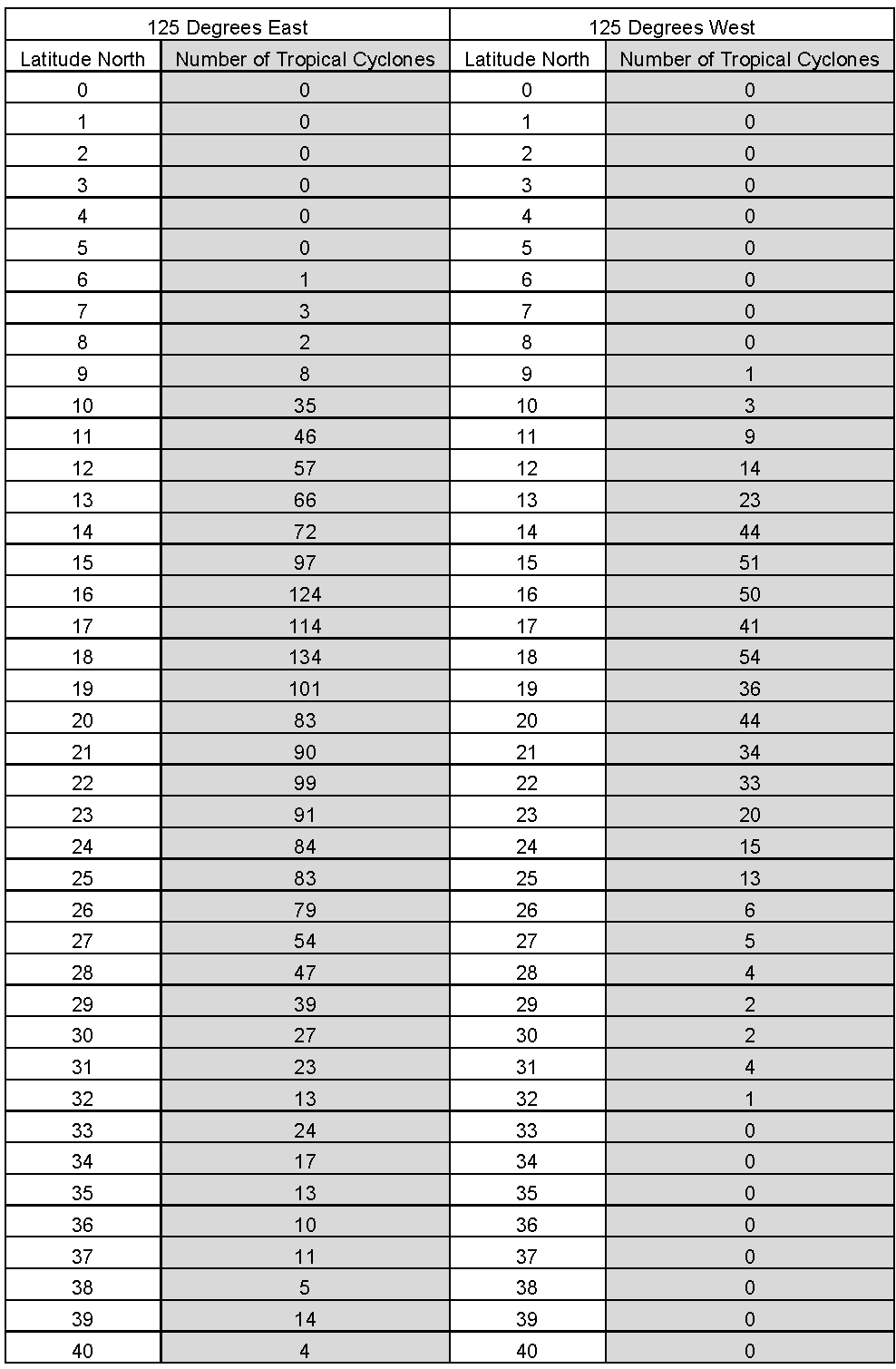## Tropical Cyclone Counts Compare Data Displays - Create Graphs

### Mini Lesson

1. Use the data table provided for the latitudes at 125° East to make a frequency table with bins that are 5 units wide.
2. Use the data table provided for the latitudes at 125° West to make a frequency table with bins that are 5 units wide.
3. What is the x axis for each histogram?
4. How many squares of the graph paper will you use for each bin?
5. What is the y axis for each histogram?
6. How much will each square of the graph paper represent?
7. How many squares will you need to graph the tallest bar? It is easier to compare if both histograms use the same scale.
8. What titles will you use?

#### Create the Histograms Using the Answers to the Planning Questions

1. Draw each set of axes using the graph paper provided.
2. Label each set of axes.
4. Using the frequency tables, draw the bars for each histogram.

1. Use the data table provided to calculate the following for the west and the east. Remember to order the data from least to greatest before doing the calculations.
2. What label will you use for each scale?
3. How many squares of the graph paper will you use for each unit?
4. How many squares will you need for each scale?
5. What titles will you use?
6. Will you draw them vertically or horizontally?

#### Create the Box plots Using the Answers to the Planning Questions

1. Draw the scale for each box plot using the graph paper provided.
2. Label the scales.
4. Using the minimum, maximum, median, lower and upper quartiles for the west and the east, draw the box plots.

1. What variable will be on the x-axis for each scatter plot?
2. What variable will be on the y-axis for each scatter plot?
3. What titles will you use?
4. How many squares of the graph paper will you use for each unit on the x-axis for each scatter plot?
5. How many squares of the graph paper will you use for each unit on the y-axis for each scatter plot? They will be easier to compare if you use the same scale for both.

#### Create the Scatter plots Using the Answers to the Planning Questions

1. Draw and label the x-axis and the y-axis for each scatter plot.
2. Label each axis on both scatter plots.
3. Add the title to each scatter plot.
4. Plot each point for each scatter plot.### Teacher Note

The purpose of this lesson is for students to create, analyze and compare histograms, box plots and scatter plots and evaluate the spread of the data. You can choose which types of graphs you want your students to complete. This is part of the Tropical Cyclone Counts Graphing Bundle and can be completed independently or with the other activities in the bundle.